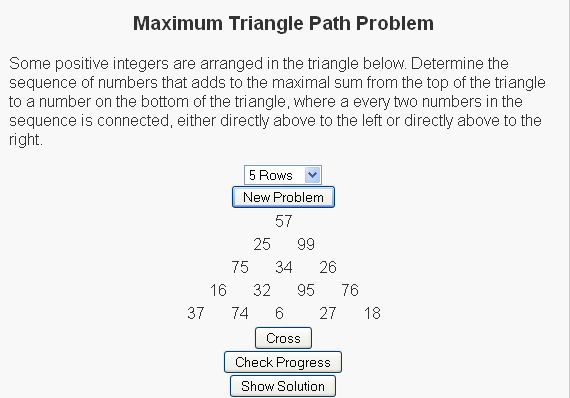# Pascal’s Triangle

I had a recent conversation with a friend who asked me “what makes number theory interesting?”. I loved the question, mainly because it gave me an opportunity to talk about math in a positive manner. More importantly though, it was an opportunity to talk about one of my favorite courses in mathematics (along with discrete mathematics and set theory). As much as the current day seems to focus on joining Number Theory with Cryptography, when I answered this question I wanted to make sure I didn’t go that route. Numbers are beautiful in their own right, and one of the things about Number Theory that was so interesting was simply the ability to look at all the different questions and patterns and properties of numbers discovered.

To answer this question, I started listing numbers to see if she noticed a pattern, but I did it with a “picture”.

.
..

….
…..
……

• How many dots will go on the next line
and
• After each line how many dots have been drawn in total?

Dots# on this line# in total
.11
..23
36
….410
…..515

There were a lot of directions I could have taken this conversation next, but I decided to stay in the realm of triangles and discuss Pascal’s triangle. This is a triangle that begins with a 1 on the first row and each number on the rows beneath is the sum of the two cells above it, assuming that cells not present have a value of zero.

So the first five rows of this triangle are

1
11
121
1331
14641

This is an interesting and beautiful triangle because of just the number of patterns you can see in it.

• Obviously there are ones on the outside cells of the triangle.
• One layer in, we get what are called the Natural or Counting numbers (1, 2, 3, 4, 5, …) .
• One layer in, we can start to see the list of numbers that I was showing my friend (1, 3, 6, 10, 15, …).

There are several other properties of this triangle and I wanted to allow users to begin to see them, so I wrote a script highlighting some of these patterns.

# Triangle Sum Puzzle

This is probably a consequence of being a mathematician, but I have always enjoyed number puzzles. I think that there is a general simplicity and universality in numbers that are not present in things like word puzzles, where the ability to reach a solution can be limited to the vocabulary of the user.

The fact that these are puzzles and not simply homework exercises also helps because we often find people sharing difficulties and successes stories over the water cooler or at the lunch table. The fact that many of these math puzzles can teach some of the same concepts as homework problems (in a more fun and inclusive way) is generally lost on the user as their primary interest is generally on solving the puzzle in front of them, or sometimes solving the more general form of the puzzle.

Today’s post is about a puzzle that was originally shared with me over a lunch table by a friend who thought it was an interesting problem and asked what I thought about it. I didn’t give the puzzle much further thought (he had correctly solved the puzzle) until I saw it again in “Algorithmic Puzzles” by Anany Levitin and Maria Levitin. It was then that I thought about the more general form of the puzzle, derived a solution for the problem, and decided to code it up as a script for my site.

Below is a link to the puzzle:We have a set of random numbers arranged in a triangle and the question is to find the path of maximum sum from the root node (the top node) to the base (one of the nodes on the bottom row) with the rules that
(1) Exactly one number must be selected from each row
(2) A number can only be selected from a row if (a) it is the root node or (b) one of the two nodes above it has been selected.

For the sample
So for the sample problem in the picture, the maximal path would go through nodes 57, 99, 34, 95, and 27.

For more of these puzzles check out the script I write here and be sure to let me know what you think.

# Triangle TrigonometryI haven’t forgotten about my pledge to focus more content here towards some of the areas I’ve been asked to tutor on recently. This latest one is designed to help users understand the properties of triangles. It is based on two laws that we learn in trigonometry: the law of sines and the law of cosines. Assume that we have a triangle with sides of lengths a, b, and c and respective angles A, B and C (where the angle A does not touch the side a, the angle B does not touch the side b, and the angle C does not touch the side c). These laws are as stated as follows:

Law of Sines

 a sin(A)
=
 b sin(B)
=
 c sin(C)

Law of Cosines
c2 = a2 + b2 – 2*a*b*cos(C)

We can use these laws to determine the sides of a triangle given almost any combination of sides and angles of that triangle (the only one we cannot determine properties from is if we are given all three angles, as this leads to many solutions).

The script generates random triangles, with different combinations of sides and angles revealed and the user’s job is to try to determine the missing sides. There is a button to reveal the solution, or if you’d like to see how we arrive at these values, you can check the “Show work” box.

Hope you enjoy.

Other Blogs covering this topic:
Mathematical!
Algebra 2 Trig